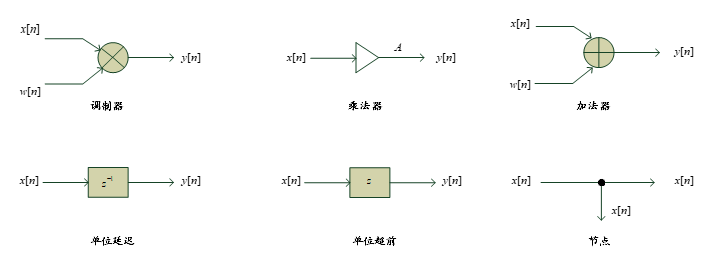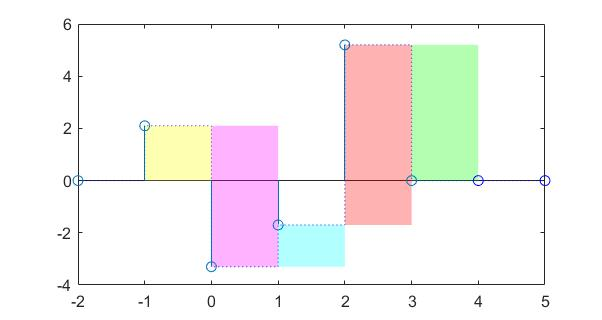# 时域中的离散时间信号

\begin{align*} \newcommand{\dif}{\mathop{}\!\mathrm{d}} \newcommand{\belowarrow}{\mathop{#1}\limits_{\uparrow}} \newcommand{\bd}{\boldsymbol} \newcommand{\L}{\mathscr{L}} \end{align*}

# 离散时间信号

## 时域描述

$x[n] = \{ \cdots, x[-1], \belowarrow{x}, x,\cdots \}$

$正变换：\bd{X}=\bd{Dx}\\ 逆变换：\bd{x}=\bd{D}^{-1}\bd{x}$

## 信号的强度

$\|x\|_p = \left( \sum_{n=-\infty}^{+\infty} \big|\, x[n]\,\big|^p \right)^{1/p}$

$p$ 常取 $1,2,\infty$。范数有一些性质，比如：$|x|_2 \leq |x|_1$，证明过程如下：

\|x\|_1 = \sum_{n=-\infty}^{+\infty} \big|\, x[n]\,\big|\\ \|x\|_2 = \sqrt{ \sum_{n=-\infty}^{+\infty} \big|\, x[n]\,\big|^2 }\\ 对两式平方：\\ \begin{align} \|x\|_1^2 &= (\cdots +|x[-1]|+|x|+|x|+\cdots)^2\\ &= \|x\|_2^2+\sum_{i\neq j} |x[i]\cdot x[j]| \end{align}

• 均方根值（rms）：$\text{rms}=\dfrac{\|x\|_2}{\sqrt{N}}$
• 均方误差（MSE）：$\text{MSE}=\frac{1}{N} (\|y[n] - x[n] \|_2)^2$
• 相对误差：$\text{E}_\text{rel} = \dfrac{\| y[n]-x[n]\|_2}{\|x[n]\|_2}$
norm(x,1) %L1范数
norm(x,2) %L2范数
norm(x,inf) %L_infinity范数


## 基本序列

### 单位样本序列 Unit Sample Sequence

$\delta[n]= \begin{cases} 1, & n=0\\ 0, & n\neq 0 \end{cases} =\{ \cdots, 0, 0, \belowarrow{1}, 0, 0, \cdots\}$
function [x,n] = impseq(n0,n1,n2)
% Generates x[n] = delta(n-n0); n1<=n<=n2

n = [n1:n2];
x = [(n-n0) == 0];

end


### 单位阶跃序列 Unit Step Sequence

$u[n]= \begin{cases} 1, & n\geq 0\\ 0, & n\lt 0 \end{cases} =\{ \cdots, 0, 0, \belowarrow{1}, 1, 1, \cdots\}$
function [x,n] = stepseq(n0,n1,n2)
% Generates x[n] = u(n-n0); n1<=n<=n2

n = [n1:n2];
x = [(n-n0)>=0];

end


### 指数序列 Exponential Sequence

$\alpha = e^{(\sigma_0+j\omega_0)}, \quad A = \vert A \vert e^{j\phi}\\ x[n] = \vert A \vert e^{j\phi} e^{(\sigma_0+j\omega_0)n}$

\begin{align} x[n] &= \vert A \vert e^{\sigma_0n} e^{j(\omega_0n+\phi) }\\ &=\vert A \vert e^{\sigma_0n} \big[ \cos(\omega_0n+\phi) + j \sin(\omega_0n+\phi)\big] \end{align}

function [x,n] = expseq(A,a,n1,n2)
% Generates x[n] = A*exp(a*n); n1<=n<=n2

n = [n1:n2];
x = A*exp(a*n);

end


### 正弦序列 Sinusoidal Sequence

$x[n] = A \cos(\omega_0n+\phi)$

$A$，$\omega_0$，$\phi$ 分别称为 振幅归一化角频率相位（准确来讲是初相位）

\begin{align} x[n] &=x_i[n]+x_q[n]\\ &=A\cos \phi \cos (\omega_0 n) - A \sin \phi \sin(\omega_n) \end{align}

>> n = [0:10];
>> x = 3*cos(2*pi*n+pi/3)


1. 若 $\omega_0 N = 2\pi r$（$N,r$ 为正整数），则为周期的。$N$ 的最小取值为基本周期
2. 在 $[0,\pi]$ 内，振荡频率随 $\omega_0$ 增加而增加，在 $[\pi,\pi]$ 内，振荡频率随 $\omega_0$ 增加而减小。$\pi$ 称为 折叠频率

### 矩形窗序列 Rectangular Window

$w_R[n] = \begin{cases} 0, & n<N_1\\ 1, & N_1 \leq n \leq N_2\\ 0, & n>N_2 \end{cases}$
function [x,n] = recwindow(n1,n2,n3,n4)
% Generates rectangular window

n = [n3:n4];
x = [n>=n1|n<=n2];

end


## 序列的运算

### 简单运算function [y,n] = sigadd(x1,n1,x2,n2)
% implements y(n) = x1(n) + x2(n)
% y = sum sequence over n, which includes n1 and n2
% x1 = first sequence over n1
% x2 = second sequence over n2
% 加法器

n = min(min(n1)，min(n2)):max(max(n1),max(n2));
y1 = zeros(1,length(n));
y2 = y1;
y1(find((n>=min(n1))&(n<=max(n1))==1))=x1;
y2(find((n>=min(n2))&(n<=max(n2))==1))=x2;
y = y1+y2;

end

function [y,n] = sigmult(x1,n1,x2,n2)
% implements y(n) = x1(n)*x2(n)
% y = product sequence over n, which includes n1 and n2
% x1 = first sequence over n1
% x2 = second sequence over n2
% 乘法器

n = min(min(n1),min(n2)):max(max(n1),max(n2));
y1 = zeros(1,length(n));
y2 = y1;
y1(find((n>=min(n1))&(n<=max(n1))==1))=x1;
y2(find((n>=min(n2))&(n<=max(n2))=x2))=x2;
y = y1.*y2

end

function [y,n] = sigshift(x,m,k)
% implement y(n) = x(n-k)
% 延时器

n = m+k;
y = x;

end

function [y,n] = sigfold(x,n)
% implements y(n) = x(-n)
% 反转

y = fliplr(x);
n = -fliplr(n);

end


### 卷积和 Convolution

$y[n] = \sum_{k-\infty}^{+\infty} x[n-k]h[k]=x[n]\circledast h[n]\\ 使用 \circledast 以免与共轭 {}^* 混淆$

function [y,ny] = conv_m(x,nx,h,nh)
%Modified convolution routine for signal processing
%  [y,ny] = conv_m(x,nx,h,nh)
%  [y,ny] = convolution result
%  [x,nx] = first signal
%  [h,nh] = second signal

nyb = nx(1)+nh(1);
nye = nx(length(x)) + nh(length(h));
ny = [nyb:nye];
y = conv(x,h);

end


### 抽样率转换

• 若 $R>1$，说明抽样率提高，该转化系统为 内插器
• 若 $R<1$，说明抽样率降低，该转化系统为 抽取器

\begin{align} &上抽样：x_u[n] = \begin{cases} x[n/L], & n=0,\pm L, \pm 2L\\ 0, &其他 \end{cases}\\ &下抽样：y[n]=x[nM] \end{align}

### 有限长序列的反转与平移

• 反转：$y[n]=x[(-n)\mod N]$ 写作 $x[\langle -n \rangle_N ]$ 1
• 平移：$y[n]=x[(n-n_0) \mod N]$ 写作 $x[\langle n-n_0 \rangle_N ]$$g[n]\,Ⓝ\,h[n] = \sum_{k=0}^{N-1} g[k]h[\langle n-k \rangle_N ]\\ 相当于：\\ \begin{bmatrix} y \\ y \\ \cdots \\y[N-1] \end{bmatrix} = \begin{bmatrix} h & h[N-1] & \cdots & h\\ h & h & \cdots & h\\ \vdots & \vdots & & \vdots\\ h[N-1] & h[N-2] & \cdots & h \end{bmatrix} \begin{bmatrix} g \\ g \\ \cdots \\g[N-1] \end{bmatrix}$

## 序列的分类

• 基于对称性

若满足 $x[n] = x^*[-n]$ ，称为 共轭对称序列

若满足 $x[n] = - x^*[-n]$ ，称为 共轭反对称序列

• 实共轭对称序列称为偶序列
• 实共轭反对称序列称为奇序列
• 任何复序列都能表示成共轭对称部分 $x_\text{cs}[n]$ 与共轭反对称部分 $x_\text{ca}[n]$ 之和，其中： $x_\text{cs}[n]=\frac{1}{2}(x[n]+x^*[-n])\\ x_\text{ca}[n]=\frac{1}{2}(x[n]-x^*[-n])$
• 周期信号非周期信号。周期信号用 $\tilde{x}[n]$ 表示
• 能量信号功率信号
• 其他序列 有界序列、绝对可和序列、平方可和序列、n点序列等，都是有某些特征的序列。

# 信号的相关

## 自相关与互相关

$\sum_{n=-\infty}^{+\infty} (x[n]-y[n-\ell])^2\\ =\sum_{n=-\infty}^{+\infty} (x^2[n]-2x[n]y[n-\ell]+y^2[n-\ell])$

$互相关：r_{xy}[\ell] = \sum_{n=-\infty}^{+\infty} x[n]y[n-\ell]\\ 自相关：r_{xx}[\ell] = \sum_{n=-\infty}^{+\infty} x[n]x[n-\ell]\\$

\begin{align} &\sum_{n=-\infty}^{+\infty} (x[n]-x[n-\ell])^2\\ =& \sum_{n=-\infty}^{+\infty} x^2[n] -2 \sum_{n=-\infty}^{+\infty} x[n]x[n-\ell] + \sum_{n=-\infty}^{+\infty} x^2 [n-\ell]\\ =& r_{xx} - 2 r_{xx}[\ell]+r_{xx}\\ \geq& 0 \end{align}\\ \therefore r_{xx}[\ell] \leq r_{xx} = \mathscr{E}_x

\begin{align} &\sum_{n=-\infty}^{+\infty} (ax[n]+y[n-\ell])^2\\ =& a^2\sum_{n=-\infty}^{+\infty} x^2[n] +2a \sum_{n=-\infty}^{+\infty} x[n]y[n-\ell] + \sum_{n=-\infty}^{+\infty} y^2 [n-\ell]\\ =& a^2 r_{xx} + 2a r_{xy}[\ell]+r_{yy}\\ \geq& 0 \end{align}\\ \therefore \begin{bmatrix} a & 1 \end{bmatrix} \begin{bmatrix} r_{xx} & r_{xy}[\ell]\\ r_{xy}[\ell] & r_{yy} \end{bmatrix} \begin{bmatrix} a \\ 1 \end{bmatrix} \geq 0\\ \Rightarrow \begin{bmatrix} r_{xx} & r_{xy}[\ell]\\ r_{xy}[\ell] & r_{yy} \end{bmatrix} 半正定\\ \therefore \begin{vmatrix} r_{xx} & r_{xy}[\ell]\\ r_{xy}[\ell] & r_{yy} \end{vmatrix} \geq 0\\ -\sqrt{r_{xx}r_{yy}} \leq r_{xy}[\ell] \leq \sqrt{r_{xx}r_{yy}}

## 相关的归一化形式

$\rho_{xx}[\ell] = \frac{r_{xx}[\ell]}{\mathscr{E}_x}\\ \rho_{xy}[\ell] = \frac{r_{xx}[\ell]}{\sqrt{\mathscr{E}_x \mathscr{E}_y}}$

# 本章练习题

2.24(a) 证明：当定义域为 $[0,+\infty)$ 时，因果实序列 $x[n]$ 可以从其偶部 $x_\text{ev}[n]$ 中完全恢复出来；而当定义域为 $(0,+\infty)$ 时，因果实序列 $x[n]$ 可以从其奇部 $x_\text{od}[n]$ 中完全恢复出来。

$$x_\text{ev}[n] = \frac{x[n]+x[-n]}{2}\\ x_\text{od}[n] = \frac{x[n]-x[-n]}{2}$$ 由前提条件，在 $(-\infty,0)$ 上 $x[n]=0$，所以有：
$$x_\text{ev}[n] = \frac{x[n]}{2},\; n>0\\ x_\text{od}[n] = \frac{x[n]}{2},\; n>0\\ \therefore x[n]=2x_\text{ev}[n]=2x_\text{od}[n],\; n>0$$ 再考虑 $x=0$ 处，显然 $x=x[-0]$，所以有：
$$x_\text{ev} = x\\ x_\text{od} = 0\quad\\$$ 综上，可以从偶部得到因果序列在 $n\geq 0$ 处的值，可以从虚部得到因果序列在 $n >0$ 处的值。证毕。

2.24(b) 证明：因果复序列 $y[n]$ 可以从其共轭对称部分 $y_\text{cs}[n]$ 中完全恢复出来；$y[n]$ 可以从其共轭反对称部分 $y_\text{ca}[n]$ 中完全恢复出来。

\begin{align} y_\text{cs}[n] &= \frac{1}{2}(y[n]+y^*[-n])\\ &=\frac{y_\text{re}[n]+y_\text{re}[-n]}{2}+j \frac{y_\text{im}[n]-y_\text{im}[-n]}{2}\\ &=y_\text{re,ev}[n]+y_\text{im,od}[n]\\ y_\text{ca}[n] &= \frac{1}{2}(y[n]-y^*[-n])\\ &=\frac{y_\text{re}[n]-y_\text{re}[-n]}{2}+j \frac{y_\text{im}[n]+y_\text{im}[-n]}{2}\\ &=y_\text{re,od}[n]+y_\text{im,ev}[n] \end{align} 根据上一题 2.24(a) 的结论：可以从偶部得到因果信号在 $n\geq 0$ 处的值，可以从虚部得到因果信号在 $n >0$ 处的值。我们可以推导出如下结论：

（1） 可以从 $y_\text{cs}[n]$ 得到 $y_\text{re}[n]$ 在 $n\geq 0$ 处的值，以及 $y_\text{im}[n]$ 在 $n>0$ 处的值。
（2） 可以从 $y_\text{ca}[n]$ 得到 $y_\text{re}[n]$ 在 $n>0$ 处的值，以及 $y_\text{im}[n]$ 在 $n\geq 0$ 处的值。

2.39(d)(e) 求以下信号的基本周期：
\begin{align} \text{(d)}\;\tilde{x}[n]&=6\sin(0.15\pi n)-\cos(0.12\pi n+0.1\pi)\\ \text{(e)}\;\tilde{x}[n]&=\sin(0.1\pi n + 0.75\pi)-3\cos(0.8\pi n+0.2\pi)+\cos(1.3\pi n) \end{align}

2.44 用单位阶跃序列 $\mu[n]$ 表示 $x[n]=\{2.1,\belowarrow{-3.3},-1.7,5.2\}$2.51 连续时间信号 $x_a(t)=\cos \Omega_o t$ 在 $t=nT$ 抽样，$-\infty\lt n\lt +\infty$，生成离散时间序列 $x[n]=x_a(nT)=\cos (\Omega_o nT)$。当 $T$ 取咩值时 $x[n]$ 是一个周期序列？若 $\Omega_o=30\text{rad}$ 且 $T=\pi/6\text{s}$，$x[n]$ 的基本周期是多少？

1. 我们用 $\langle n \rangle_N$ 表示 $n \mod N$ ，某些教材（比如 Oppenheim 的教材）也将取模运算写作：$((n))_N$